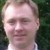# Sampling Poser

ByRobin Barnwell

I thought I would share my views on a question frequently posed by newly trained belts. I imagine you to may have encountered this situation. I do not have a clear answer but have come-up with a theory. Could be right, could be wrong.

We talk about the discrete sampling equation used to calculate minimum sample size

Minimum Sample Size = Square of (1.96 / Precision) * Est. Proportion * (1 – Est. Proportion)

For example, what is the sample size required to find, within 5%, the number of people who are left-handed using a starting assumption that 10% of the population are left-handed?

Minimum Sample Size = Square of (1.96 / 0.05) * 0.1 * (1 – 0.1) = 138

But what people ask is, what if there are more than two categorise? What if you want to know the sample size required to find the proportion of calls split into:

• Renewal Quotes
• Change of Service Quotes

Now I haven’t been able to find much of an answer to this question. I have come-up with a theory but I do not think it is statistically robust. Interested on comments and if there is an off-the-shelf statistical solution I have missed and can apply:

• Build an exploratory sample
-Start by assuming each category is equally weighted. So the estimated proportion for each is 25%. Using quite a wide precision (e.g. 10%) you get the sample size of 72.
-To allow for the extra categorise multiple by thetotal number and divide by two, hence 72 * 4 / 2, to give a final sample size of 144. The result gives you a “feel for the proportions” but is by no means accurate.
• Develop the proportions
-You now have a feel for the proportions e.g. 60%, 30%, 10%, & 10%.
-Because sampling theory says that 50% proportions require the highest sample size use the proportion nearest to 50%. In this case the 60% one.
-Calculate your sample size based on 60% so using 5% precision you get 369
-Including theextra factor to allow for multiple categorise you get 369 * 4 /2, to give a final sample size of 738
-You can then find your confidence interval from the results obtains
Handpicked Content:   Don't Bury the Past

I have made-up this approach and have no idea if it will stand-up to scrutiny. Hopefully I am on the right-track.

You never know it might become true like 1.5 sigma-shift……

##### Robin Barnwell

Financial Services

1.Robert-Butler

You can run power calculations for more than two categories. I don’t happen to know the mathematical expressions needed for this effort but a number of the more advanced statistical packages have this capability. In SAS under Proc Power is the option for ONEWAYANOVA which generates sample estimates for multiple categories given their means and an estimate of pooled variation. However, as far as I know it won’t handle multiple proportions.

2.Robin Barnwell

Hello Robert

Thanks for the update. Yes, it’s the multiple proportion sampling that’s of interest. I am investigating if there is a sampling plan for Chi-Square. This might well solve the problem…..

Robin

3.Robin Barnwell

Just a quick update on this problem – I have solved it!

Its based on sample planning using the chi-square distributions. The answer is quite straight-forward once you can see how it work.

This answers the perennial training question; how many do you need to sample for a multi- modal distribution e.g. defects by type.